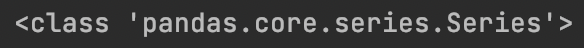• VBA源代码，根据某中的最大值，拿到该行的行号。此代码为取出若干小区的信息，7*24小时，拿到某值最大的行号，精简成7行
• rw.Cells(1, 11).FormulaArray = “=MAX(IF(打印记录!K:K=Sheet1!J” & rw.Row & “,打印记录!L:L))”

rw.Cells(1, 11).FormulaArray = “=MAX(IF(打印记录!K:K=Sheet1!J” & rw.Row & “,打印记录!L:L))”

展开全文python java 算法
• 如何剪裁csv文件，并从中出每一列最大值和平均值，并将最大值和平均值添加到表的末尾剪裁CSV文件一列最大值和平均值将最大值和平均值添加到末尾 很少用python，第一次用python来处理csv文件，算是一个从无...


如何剪裁csv文件，并从中求出每一列的最大值和平均值，并将最大值和平均值添加到表的末尾
剪裁CSV文件求每一列的最大值和平均值将最大值和平均值添加到末尾

很少用python，第一次用python来处理csv文件，算是一个从无到有的过程吧，文中诸多地方可能有错，感谢指正！

剪裁CSV文件
# 按需求读取csv文件
"""
参数说明：
# csv_path是我需要被pandas读取的文件路径
# usecols是我需要的列，可以指定其具体名称，也可以以数组的形式指定，0即代表第一列
# skiprows是指需要跳过的行，注意这不是删除前几行，skip的行数超过属性行会导致读取报错
# encoding是指定文件的编码方式，防止读取出来的文件产生乱码
# index_col一共可以指定三个：None/0/False，详情请参照：https://blog.csdn.net/weixin_40992494/article/details/104535719
# nrows是需要读取的行数
"""

df = pd.read_csv(csv_path, usecols=[0,1,2,3], skiprows=2, encoding='utf8',
index_col=0, nrows=80)


读取到了之后并不会对原文件进行更改，最后要将更改的结果导出，我需要导出一个csv文件，故使用
df.to_csv('这里填需要导出的路径')

求每一列的最大值和平均值
其实一开始挺懵逼的，看起来很简单的东西，也走了一些弯路。。。 首先需要了解到，使用pandas求最大值和平均值需要使用的是df.max()和df.mean()。 在上面使用read()的时候我已经指定了index_col=0，即第一行是index，其本身是不会参与计算的（这正和我意）但是傻傻的使用了loc去规避第一行。。。 记录一下错误的方法： 首先写了一个list指定了需要计算平均值和最大值的cols（其实就是除了第一列以外的。。） 然后使用了loc函数：
'''
round()是保留小数点后几位
'''
mean = df.loc[:, col_list].mean().round(2)
max = df.loc[:, col_list].max().round(2)

我认为正确的做法：
mean = df.mean().round(2)
max = df.max().round(2)

因为我取到的数据已经是我想要的列了，然后第一列也是不需要参与计算的index，所以就这样就能得到正确的结果了
将最大值和平均值添加到末尾
添加数据到dataframe，经过我在网上搜索最终决定用append()，但是在添加之前我们需要了解到刚才计算出的结果到底是什么格式，将上面得到的mean和max数据格式打印出来：是series形式的数据，把两个结果拼好然后append进原表就好了。需要注意的是，得到的series是没有index的，所以需要为其添加一个name：
series_0 = pd.Series(max, name="最大值")
series_1 = pd.Series(mean, name="平均值")
# 合并
series = pd.concat([series_0, series_1], axis=1)

然后再使用append添加即可：
'''
T很关键，可以将series转置，不转置的话会导致插入错误，因为得到的数据格式将原本的col作为了index
'''
df = df.append(series.T)

最后记得to_csv()导出到文件 这部分知识是吃的快餐，理论上肯定有所纰漏，但结果是没错的，望指正！
展开全文• 取出很多小区的数据，比如说有7行，根据某列值排序取出前4行的，然后每按照这个平均。VBA通信领域的实际应用。
• 拿wps举例，MS的Excel也有相应功能。...第三步，输入最大值最小值公式即可。（注意公式=A2，一定是要从你数据开始的单元格来写） 成果展示 当然了，这只是查找，所以对同样的数据不防呆。 （每行同样适用） ...
拿wps举例，MS的Excel也有相应功能。
用到的功能：格式条件第一步，选中你想查找的数据区域 第二步，打开格式条件（格式→格式条件→新建规则→如图）第三步，输入最大值最小值公式即可。（注意公式=A2，一定是要从你数据开始的单元格来写）成果展示当然了，这只是查找，所以对同样的数据不防呆。 （每一行同样适用）
展开全文excel
• '汇总每个sheet的结果,最大值,最小值之类的 Dim arr, dic, colh, rowh Dim i%, j%, k% Dim rmax(), rmin(), rrange(), rmaxamongpulley(), rminamongpulley(), rmaxamongeachrun(), rminamongeachrun()...

Sub sum_table()
'汇总每个sheet的结果,最大值,最小值之类的
Dim arr, dic, colh, rowh
Dim i%, j%, k%
Dim rmax(), rmin(), rrange(), rmaxamongpulley(), rminamongpulley(), rmaxamongeachrun(), rminamongeachrun() As Double
Dim coln, rown As Integer, n As Integer, m As Integer

'ActiveSheet.name = "sum"

n = Worksheets.Count
For k = 3 To n
g = Worksheets("content").Cells(k - 2, 1).Value
coln = Worksheets(g).UsedRange.Columns.Count
coln = Worksheets(g).[IV1].End(xlToLeft).Column
rown = Worksheets(g).UsedRange.Rows.Count

colnsum = Worksheets("sum").[IV1].End(xlToLeft).Column + 1

'取列头,并去重
Set dic = CreateObject("scripting.dictionary")
Worksheets(g).Activate
arr = ActiveSheet.Range(Cells(2, 1), Cells(2, coln))
For i = 1 To UBound(arr)
For j = 1 To UBound(arr, 2)
dic(arr(i, j)) = ""
Next j
Next i
rowh = Application.Transpose(dic.keys)

Set dic = Nothing
'取行头,并去重
Set dic = CreateObject("scripting.dictionary")
Worksheets(g).Activate
arr = ActiveSheet.Range(Cells(3, 1), Cells(3, coln))
For i = 1 To UBound(arr)
For j = 1 To UBound(arr, 2)
dic(arr(i, j)) = ""
Next j
Next i
colh = dic.keys

Worksheets("sum").Activate
Range(Cells(2 + 1 * (UBound(rowh) + 1), colnsum).Address).Resize(UBound(rowh), 1) = rowh
Range(Cells(2 + 2 * (UBound(rowh) + 1), colnsum).Address).Resize(UBound(rowh), 1) = rowh

Range(Cells(1 + 0 * (UBound(rowh) + 1), 1 + colnsum).Address).Resize(1, UBound(colh) + 1) = colh
Range(Cells(1 + 1 * (UBound(rowh) + 1), 1 + colnsum).Address).Resize(1, UBound(colh) + 1) = colh
Range(Cells(1 + 2 * (UBound(rowh) + 1), 1 + colnsum).Address).Resize(1, UBound(colh) + 1) = colh

Set dic = Nothing
'根据行头,列头取最大值
ReDim rmax(1 To UBound(rowh), 1 To UBound(colh) + 1)
ReDim rmin(1 To UBound(rowh), 1 To UBound(colh) + 1)
ReDim rrange(1 To UBound(rowh), 1 To UBound(colh) + 1)
ReDim Preserve rmaxamongpulley(1 To n - 2, 1 To UBound(colh) + 1)
ReDim Preserve rminamongpulley(1 To n - 2, 1 To UBound(colh) + 1)

Worksheets(g).Activate
For i = 0 To UBound(colh)
For j = 1 To UBound(rowh)
For m = 1 To coln
If colh(i) = Worksheets(g).Cells(3, m).Value And rowh(j, 1) = Worksheets(g).Cells(2, m).Value Then
rmax(j, i + 1) = WorksheetFunction.Max(ActiveSheet.Range(Cells(5, m), Cells(rown, m)))
rmin(j, i + 1) = WorksheetFunction.Min(ActiveSheet.Range(Cells(5, m), Cells(rown, m)))
rrange(j, i + 1) = rmax(j, i + 1) - rmin(j, i + 1)
End If
Next
Next
rmaxamongpulley(k - 2, i + 1) = WorksheetFunction.Max(WorksheetFunction.Index(rmax, 0, i + 1))
rminamongpulley(k - 2, i + 1) = WorksheetFunction.Min(WorksheetFunction.Index(rmin, 0, i + 1))
Next

Worksheets("sum").Activate
Range(Cells(2 + 0 * (UBound(rowh) + 1), 1 + colnsum).Address).Resize(UBound(rowh), UBound(colh) + 1) = rmax
Range(Cells(2 + 1 * (UBound(rowh) + 1), 1 + colnsum).Address).Resize(UBound(rowh), UBound(colh) + 1) = rmin
Range(Cells(2 + 2 * (UBound(rowh) + 1), 1 + colnsum).Address).Resize(UBound(rowh), UBound(colh) + 1) = rrange

Worksheets("sum").Cells(1 + 0 * (UBound(rowh) + 1), colnsum).Value = g & "-max"
Worksheets("sum").Cells(1 + 1 * (UBound(rowh) + 1), colnsum).Value = g & "-min"
Worksheets("sum").Cells(1 + 2 * (UBound(rowh) + 1), colnsum).Value = g & "-range"
Next

ReDim rmaxamongeachrun(1 To UBound(colh) + 1)
ReDim rminamongeachrun(1 To UBound(colh) + 1)
For i = 1 To UBound(rmaxamongpulley, 2)
rmaxamongeachrun(i) = WorksheetFunction.Max(WorksheetFunction.Index(rmaxamongpulley, 0, i))
rminamongeachrun(i) = WorksheetFunction.Min(WorksheetFunction.Index(rminamongpulley, 0, i))
Next

Worksheets("sum").Activate
For k = 3 To n
g = Worksheets("content").Cells(k - 2, 1).Value
Range(Cells(2 + 3 * (UBound(rowh) + 1) + k - 3, 2).Address).Resize(1, 1) = g
Range(Cells(2 + 3 * (UBound(rowh) + 1) + k - 3 + n, 2).Address).Resize(1, 1) = g
Next

Range(Cells(1 + 3 * (UBound(rowh) + 1), 3).Address).Resize(1, UBound(colh) + 1) = colh
Range(Cells(1 + 3 * (UBound(rowh) + 1) + n, 3).Address).Resize(1, UBound(colh) + 1) = colh

Range(Cells(2 + 3 * (UBound(rowh) + 1), 3).Address).Resize(UBound(rmaxamongpulley), UBound(rmaxamongpulley, 2)) = rmaxamongpulley
Range(Cells(2 + 3 * (UBound(rowh) + 1) + n, 3).Address).Resize(UBound(rminamongpulley), UBound(rminamongpulley, 2)) = rminamongpulley
Range(Cells(3 + 5 * (UBound(rowh) + 1), 3).Address).Resize(1, UBound(rmaxamongeachrun)) = rmaxamongeachrun
Range(Cells(4 + 5 * (UBound(rowh) + 1), 3).Address).Resize(1, UBound(rminamongeachrun)) = rminamongeachrun

Worksheets("sum").Cells(1 + 3 * (UBound(rowh) + 1), 2).Value = "max among pulley"
Worksheets("sum").Cells(1 + 3 * (UBound(rowh) + 1) + n, 2).Value = "min among pulley"
Worksheets("sum").Cells(4 * (UBound(rowh) + 1), 2).Value = "max among run"
Worksheets("sum").Cells(4 * (UBound(rowh) + 1) + n, 2).Value = "min among run"

End Sub

展开全文excel
• Debug.Print Worksheets(1).[A65536].End(xlUp).Row ...功能是返回个 Range 对象，该对象代表包含源区域的区域尾端的单元格。等同于按键 End+ 向上键、End+ 向下键、End+ 向左键或 End+ 向右键。Range 对象，只读。
• 前言：因为考虑数据量的问题，所以在计算季度数据的时候，做了个辅助“季度累计数（至上月）”，季度累计数=本月发展数+季度累计数（至上月），这样就碰到个问题：需要每个月都把季度累计数复制到季度累计数...
• 前言：之前在使用工作簿与工作簿之间的数据连接时，使用的是vlookup手动做链接，然后断开连接并另存为的方式，虽然做好次后很方便，但是每次变动需求要修改的话都好累。比如我这里有36个，也就是相当于要做36次...
• 此算法是从一列数据中去掉最大数值和最小数值,出总和,平均值,计算出  在cell中填充去掉的最大值,最小值, 总和,平均值和方差.  算法效率有点低,主要解决业务问题了. Public Sub aver()  Application....
• =MATCH(MAX(N4511:N9019),N4511:N9019,0)
• 然后获取每组的value最大值和最小值。因此最终结果，我希望获得如下  group, maxvalue, minvalue 1, 15, 11 2, 23, 12 3, 23, 2  我在想如何不用 游标或者循环遍历表，来获得这样的结果？ 想了很...sql
• 暂时只实现了删除一列中的空行，并没有实现多行的判断空行和删除方法。----之后再做更复杂的 1.1需求分析 用VBA删除如下内容，解决思路都不同 删除1列的空行（本文要做的） 删除整个区域内的空行 删除整个...
• VBA对Excel文件进行处理的时候，关键字段的号编程时往往是不知道的，需要通过参数设定才能知道，因此，我们编程的时候，就不能用这样的语句取有效行数： lineno = [B65536].End(xlUp).Row  上述语句中的列名“B...
• 大家好，我们今日继续讲解VBA数组与字典解决方案第22讲：在VBA中如何使用动态数组，以及利用动态数组去除重复的方法。如果文本中含有大量的重复，此时，如果我们要剔除重复，该怎么办？用VBA的方法该如何做到...
• 在Excel中，经常需要得知某最后个非空单元格的行号，以确定数据的数量。编写自定义函数以实时计算某单元格所在的最后非空单元格的行号。 员工编号 姓名 部门 0114 俞卫广 总经办 0374 ...
• 注意-有个附加的“ VBA分析财务数据bonus.bas”脚本，其中包括上述内容以及以下内容：最大百分比增长，最大百分比下降和最大总交易量。 还将重复浏览Excel文件中的每个工作表 特征 VBA脚本执行以下操作： 遍历...
• 方法 读/写 类别 默认值 说明 .ColumnGrand ...是否启用汇总 ...数据透视表在刷新或移动域时自动设置...如果数据透视表在有错误的单元格中显示用户自定义的错误字符串，则该为 True。 .ErrorString 返回...excel
• 代码中：个函数负责获取每个表的有效记录最大行数，个函数负责填充公式。调用的Sub是个应用实例。 使用前建议用录制宏获得需要填充的公式。 本人在个测试用例excel-word转换简易工具中应用这个实现记录完整...
• 函数作用:将工作表中最后一列作为页脚打印在每一面页尾101 '102.函数作用:获取vbproject引用项目.................102 '103.函数作用:移除Excel工作表中的外部数据连接.......103 '104.函数作用:将选择定单元格作成...
• ## excel vba数据分析

千次阅读 2019-01-20 12:20:00
VBA(Visual Basic for Application)是Microsoft Office系列软件的内置编程语言，其语法结构与Visual Basic编程语言互相兼容，采用的是面向对象的编程机制和可视化的编程环境。 第节 标识符 ．定义 标识符是...
• Excel数据处理1 隔行取数1.1 隔行取数后取最大值 1 隔行取数 问题：Excel中每隔10个数取个值。 方法：OFFSET或INDEX函数。 OFFSET(reference;rows;cols;[height];[width]) OFFSET（基准位置，向下或上偏移几行，...
• 使用VBA添加ListObject对象 Excel中数据存储的结构 (1)用输入界面的表格或者汇总形式的表格直接代替数据库存储。 (2)使用多维表（交叉表）记录数据。 示例 如图所示，该表为某公司供应商信息录入表以及供应商...
• EXCEL随机数据 使用 单元格表达式 以及 Excel VBA 快速生成随机数据。使用单元格表达式（函数）在指定范围产生随机整数、小数、大小写英文字符、字符串、随机日期和随机时间，并设定输出的时间格式和日期格式。产生...excel
• Excel VBA程序，功能包括： 1. 转换格式：将一个目录内多个txt文件，分别转换成同名的excel文件并保存。 2. 汇总数据：读取一个目录内每个Excel文件中的...3. 数据统计：统计一列数据的最小值、最大值、平均值、标准差
• 新建个.xlsm文件（打开.xlsx源数据文件并另存为.xlsm也可以），输入数据源（源数据和VB代码可以从以下链接下载）： 打开.xlsm文件后在当前Sheet的名字上右击，选“View Code”（或者直接快捷键Alt+F11打开VB...折线图 查找 excel
• _Worksheet ws; Range range; range ＝ ws.GetUsedRange();//获得Worksheet已使用的范围 ...range = range.GetRows(); //获得总行数（LPDISPATCH类型） ...获得数也是一样的方法，把GetRow
• 1 MsgBox Val(Workbooks(1).Worksheets(1).Range("b:b").Find("*", , , , , xlPrevious).Row)'查找最大行号 2 3 MsgBox Val(Workbooks(1).Worksheets(1).Range("b:b").Find("*", , , , , xlPrevious).column)'......